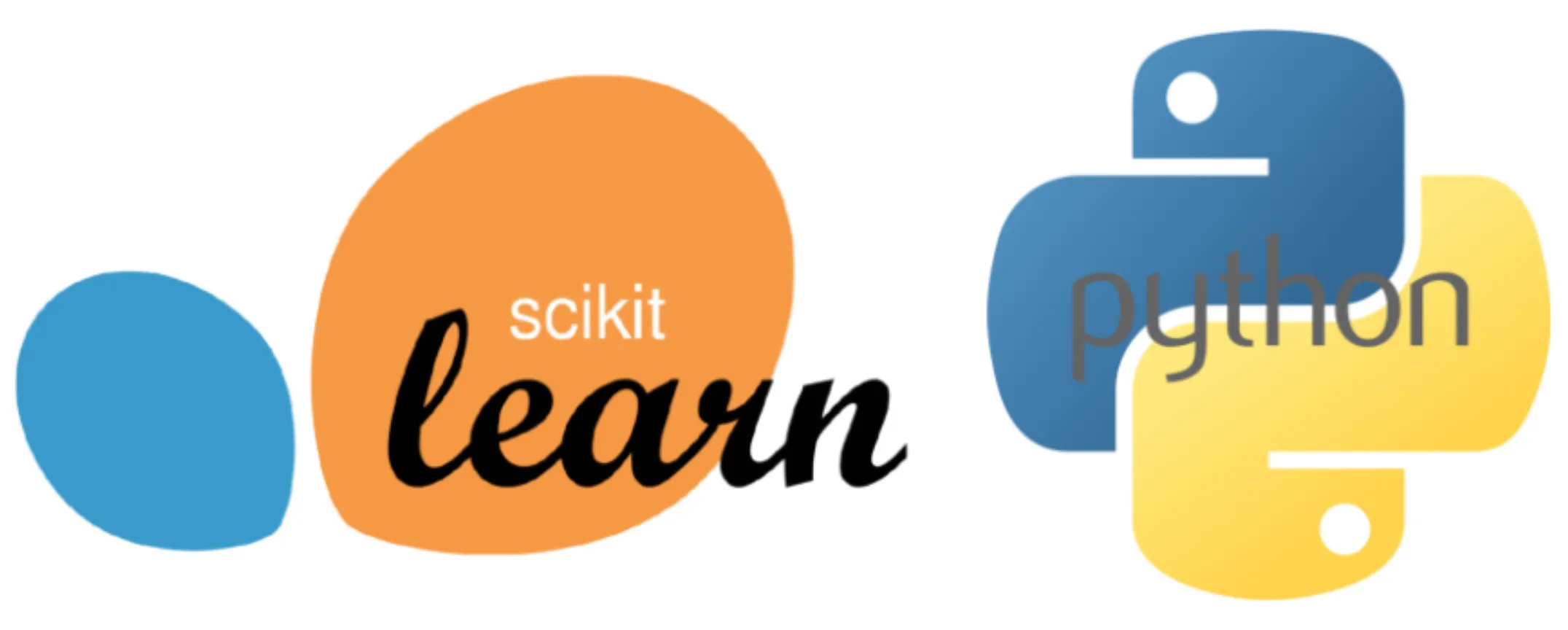# A Comprehensive Introduction to Scikit-Learn: A Key Python Machine Learning Library# A Comprehensive Introduction to Scikit-Learn: A Key Python Machine Learning Library

Machine learning is a rapidly evolving field, and Python has emerged as a significant language due to its simplicity and the availability of robust libraries designed for machine learning. One such library is Scikit-learn, a versatile and efficient tool that provides a wide range of supervised and unsupervised learning algorithms. This comprehensive guide will introduce you to Scikit-learn, its features, and how to use it effectively in your machine learning projects.

## What is Scikit-learn?

Scikit-learn is a Python library that provides a range of supervised and unsupervised learning algorithms via a consistent interface. It is built upon the SciPy (Scientific Python) stack, which must be installed before you can use Scikit-learn. This stack includes NumPy for n-dimensional array package, SciPy for scientific computing, Matplotlib for comprehensive 2D/3D plotting, IPython for an enhanced interactive console, Sympy for symbolic mathematics, and Pandas for data structures and analysis.

The library is licensed under a permissive simplified BSD license and is distributed under many Linux distributions, encouraging academic and commercial use. The vision for the library is a level of robustness and support required for use in production systems. This means a deep focus on concerns such as ease of use, code quality, collaboration, documentation, and performance.

## Features of Scikit-learn

Scikit-learn is focused on modeling data. It is not focused on loading, manipulating, and summarizing data. For these features, refer to NumPy and Pandas. Some popular groups of models provided by Scikit-learn include:

– Clustering: for grouping unlabeled data such as KMeans.
– Cross Validation: for estimating the performance of supervised models on unseen data.
– Datasets: for test datasets and for generating datasets with specific properties for investigating model behavior.
– Dimensionality Reduction: for reducing the number of attributes in data for summarization, visualization, and feature selection such as Principal component analysis.
– Ensemble methods: for combining the predictions of multiple supervised models.
– Feature extraction: for defining attributes in image and text data.
– Feature selection: for identifying meaningful attributes from which to create supervised models.
– Parameter Tuning: for getting the most out of supervised models.
– Manifold Learning: For summarizing and depicting complex multi-dimensional data.
– Supervised Models: a vast array not limited to generalized linear models, discriminate analysis, naive bayes, lazy methods, neural networks, support vector machines, and decision trees.

## Using Scikit-learn: An Example

To illustrate how easy it is to use Scikit-learn, let’s consider an example where we use the Classification and Regression Trees (CART) decision tree algorithm to model the Iris flower dataset. This dataset is provided as an example dataset with the library and is loaded. The classifier is fit on the data, and then predictions are made on the training data. Finally, the classification accuracy and a confusion matrix is printed.

## Relevant Prompts for Understanding Scikit-learn

To help you get started with understanding Scikit-learn, here are some prompts that you can use:

1. “What is Scikit-learn and why is it important in machine learning?”
2. “What are the key features of Scikit-learn?”
3. “How does Scikit-learn support both supervised and unsupervised learning algorithms?”
4. “What is the role of the SciPy stack in Scikit-learn?”
5. “How can Scikit-learn be used for clustering in machine learning?”
6. “How does Scikit-learn support cross-validation in machine learning models?”
7. “How can Scikit-learn be used for dimensionality reduction in machine learning?”
8. “What are the ensemble methods supported by Scikit-learn?”
9. “How can Scikit-learn be used for feature extraction in machine learning?”
10. “How can Scikit-learn be used for parameter tuning in machine learning models?”

In conclusion, Scikit-learn is a powerful, versatile, and efficient Python library for machine learning. It provides a wide range of supervised and unsupervised learning algorithms, making it an essential tool for any machine learning practitioner. By understanding and effectively using Scikit-learn, you can significantly enhance your machine learning projects.

# Personal Career & Learning Guide for Data Analyst, Data Engineer and Data Scientist

## Applied Machine Learning & Data Science Projects and Coding Recipes for Beginners

A list of FREE programming examples together with eTutorials & eBooks @ SETScholars

# Projects and Coding Recipes, eTutorials and eBooks: The best All-in-One resources for Data Analyst, Data Scientist, Machine Learning Engineer and Software Developer

Topics included:Classification, Clustering, Regression, Forecasting, Algorithms, Data Structures, Data Analytics & Data Science, Deep Learning, Machine Learning, Programming Languages and Software Tools & Packages.
(Discount is valid for limited time only)## Find more … …

Machine Learning for Beginners – A Guide to Compare Machine Learning Algorithms in Python with scikit-learn

Machine Learning for Beginners in Python: Linear Regression Using Scikit-Learn

Regression Machine Learning Algorithms in Python with scikit-learn